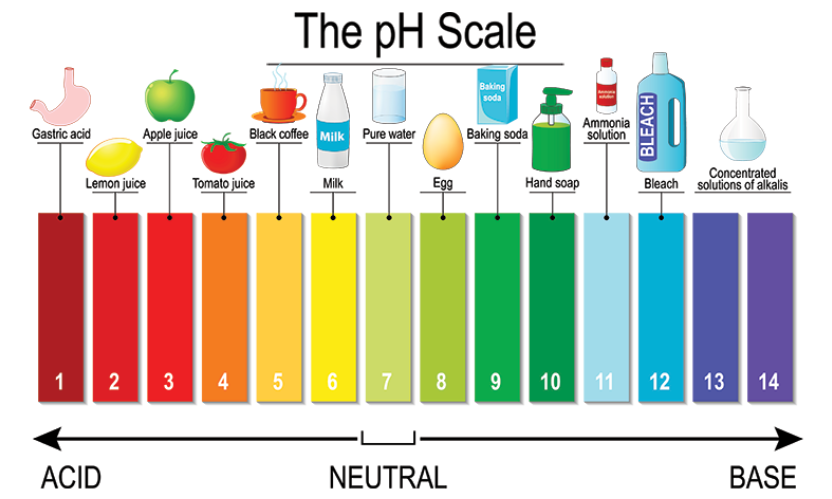# On heating blue coloured powder of copper (II) nitrate in a boiling tube, copper oxide (black), oxygen gas and a brown gas X is formed(a) Write a balanced chemical equation of the reaction.(b) Identity the brown gas X evolved.(c) Identify the type of reaction.(d) What could be the pH range of aqueous solution of the gas X?

(a) The balanced equation is given below:
$2Cu (NO_{3})_{2}(s)\overset{heat}{\rightarrow} 2CuO(s)+4NO_{2}(g)+O_{2}(g)$\

(b) The brown gas X= NO2 (Nitrogen dioxide) is released.

(c) On heating blue coloured powder of copper (II) nitrate in a boiling tube, black copper oxide, O2, and a brown gas X is formed. Hence, it is a Decomposition Reaction.

(d) pH range of aqueous solution of the gas X= NO2 is between 0 to 7 i.e., acidic pH range, NO2 when dissolved in water gives Nitric acid (HNO3).

(Refer image for better understanding)The pH of a solution is a measure of the molar concentration of hydrogen ions in the solution and as such is a measure of the acidity or basicity of the solution.

The letters pH stand for "power of hydrogen" and the numerical value for pH is just the negative of the power of 10 of the molar concentration of H+ ions.)